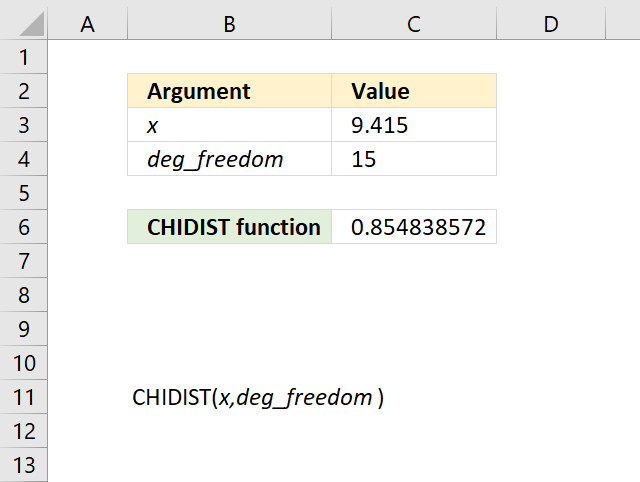Author: Oscar Cronquist Article last updated on May 11, 2022The CHIDIST function calculates the probability of the chi-squared distribution. Use this function to check if a hypothesize is valid.

The CHIDIST function is outdated and you will find it in the compatibility category, the function has been replaced by the CHISQ.DIST function and the CHISQ.DIST.RT function.

Formula in cell C6:

=CHDIST(C3,C4)

### Excel Function Syntax

CHIDIST(x,deg_freedom)

### Arguments

 x Required. A numerical value representing a point in the probability distribution you want to be evaluated. deg_freedom Required. A numerical value representing the degrees of freedom.